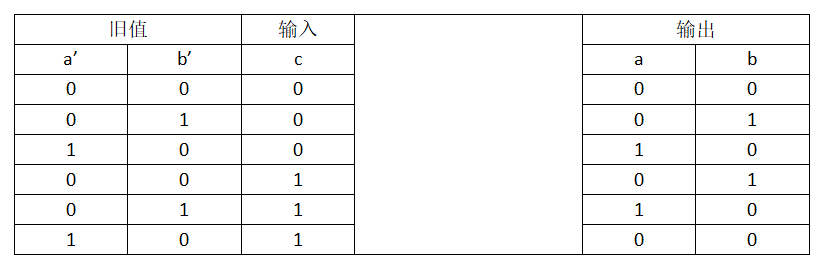2019/04/10 10:10

# 题解：

    public int singleNumber(int[] nums) {
//统计各个数字出现的次数，键为数字，值为出现的次数
Map<Integer,Integer> map =new HashMap<Integer,Integer>();
for(int i:nums){
if(!map.containsKey(i)){
map.put(i,1);
continue;
}
map.put(i,map.get(i)+1);
}
//遍历map中的键值对，查看值出现次数为1的键，即为答案
int result = 0;
for(Map.Entry<Integer,Integer> entry:map.entrySet()){
if(entry.getValue()==1){
result = entry.getKey();
break;
}
}
return result;
}


    public int singleNumber(int[] nums) {
if(nums==null||nums.length==0) return 0;
int result = 0;
for(int i = 0;i<32;i++){
//统计该位1的出现次数情况
int count = 0;
int index = 1<<i;
for(int j:nums){
//该位与操作后的结果不为0，则表示该位为1的情况出现了
if((index&j)!=0){
count++;
}
}
//该位上出现1的次数mod3后为1，表示出现一次的数字该位为1
if(count%3==1){
result|=index;
}
}
return result;
}public int singleNumber(int[] nums) {
//a对应位为1表示出现2次的记录，b对应位表示出现1次或0次的记录，ab共同组成该位出现的次数
int a = 0,b =0;
for(int i:nums){
int temp = a;
a = (~a&b&i)|(a&~b&~i);
b = ~temp&(b^i);
}
return b;
}


    public int singleNumber(int[] nums) {
//a为对应位的1出现2次的记录，b为对应位出现1次的记录，ab共同组成该位出现的次数
int a = 0,b =0;
for(int i:nums){
b = ~a&(b^i);
a = ~b&(a^i);
}
return b;
}0
0 收藏

### 作者的其它热门文章0 评论
0 收藏
0